# Static vs Non-Static Members in C#

## Static vs Non-Static Members in C# with Examples

In this article, I am going to discuss the Static vs Non-Static Members in C# with Examples. Please read our previous article before proceeding to this article where we discussed the Data Types in C# with Examples. At the end of this article, you will be having a very good understanding of the following pointers.

1. What are Static and Non-Static members in C#?
2. When do we need to use Static and Non-Static Members in C#?
3. Static and Non-static Variables in C#.
4. What is the scope of Non-Static Variables in C#?
5. Static and Non-Static Methods in C#.
6. What are Static and Non-Static Constructors in C#?
7. Understanding Static Class in C#.
##### What are Static and Non-Static Members in C#?

The member of a class is divided into two categories

1. Static Members
2. Non-Static Members

The members (Variables, Constructors, and Methods) which are created by using the static modifier are called static members rest of all are called non-static members.

In other words, we can also define that, the members of a class that does not require an instance either for initialization or execution are known as the static member. On the other hand, the members which require an instance of a class for both initialization and execution are known as non-static members.

##### Understanding Static and Non-Static Variables in C#

Whenever we declare a variable by using the static modifier or when we declare a variable inside of any static block then those variables are considered static variables whereas the rest of the others are considered non-static variables.

For a better understanding, please have a look at the following code. In the below example, variable y is static and variable x is non-static. Again, variable a is declared inside a static block, and hence variable a is by default static but it is a local variable. Similarly, variable b is declared inside a non-static block and hence it is a non-static variable but it is a local variable.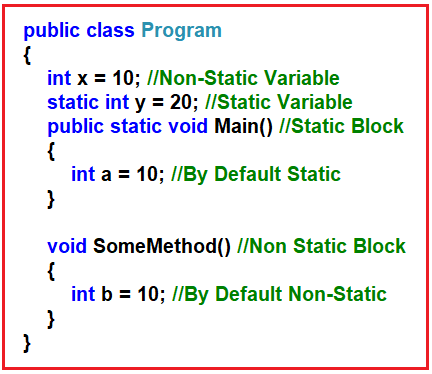If you want a variable to have the same value throughout all instances of a class then you need to declare that variable as a static variable. So, the static variables are going to hold the application-level data which is going to be the same for all the objects.

The static variable gets initialized immediately once the execution of the class starts whereas the non-static variables are initialized only after creating the object of the class and that is too for each time the object of the class is created.

A static variable gets initialized only once during the life cycle of a class whereas a non-static variable gets initialized either 0 or n number of times, depending on the number of objects created for that class.

If you want to access the static members of a class, then you need to access them directly within the same class and use the class name from outside the class whereas you need an instance of a class to access the non-static members.

##### Example to Understand Static and Non-Static Variables in C#

Let us see an example for a better understanding of the static and non-static variables in C#. Please have a look at the below example. Here, we created two variables one is static (i.e. static int y = 200;) and one non-static variable (i.e. int x;). Then using the constructor of the class, we initialize the non-static variable. Further, if you look at the Main method, we are accessing the static variable using both class name and directly (as accessing the static variable within the same class) and for accessing the non-static variable we created two instances of the class.

```using System;
namespace StaticNonStaticDemo
{
class Example
{
int x; // Non-Static Variable
static int y = 200; //Static Variable
public Example(int x)
{
//Initializing Non-Static Variable
this.x = x;
}
static void Main(string[] args)
{
//Accessing Static Variable using class name
//Before Object Creation
Console.WriteLine(\$"Static Variable Y = {Example.y}");

//Accessing Static Variable using without class name
//It’s possible because we are accessing the variable
//from the same class
Console.WriteLine(\$"Static Variable Y = {y}");

//Creating Object1
Example obj1 = new Example(50);

//Creating Object2
Example obj2 = new Example(100);

//Accessing Non-Static Variable using Object of the class
Console.WriteLine(\$"Object1 x = {obj1.x} and Object2 x = {obj2.x}");
}
}
}
```
###### Output: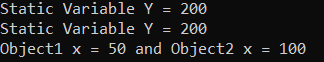##### What is the Scope of Static and Non-Static Variables in C#?

The Non-Static variables are created when the object is created and are destroyed when the object is destroyed. The object is destroyed when its reference variable is destroyed or initialized with null. So, we can say that the scope of the object is the scope of its referenced variables. On the other hand, the Static Variable scope is the Application Scope. What it means, as soon as the application start and class execution starts, static variables are created and they will be there as long as your application is running. Once the application stops, then static variables are going to be deleted. So, the scope is application scope.

##### Static and Non-Static Methods in C#

When we create a method by using the static modifier then it is called the static method and the rest of the methods are non-static methods. You cannot consume the non-static members directly within a static method. If you want to consume any non-static members with a static method then you need to create an object and then through the object, you can access the non-static members. On the other hand, you can directly consume the static members within a non-static method without any restriction.

##### Rules to follow while working with Static and Non-Static Members in c#:
1. Non-Static to Static: Non-Static Members can be consumed only by using the object of that class inside a static block.
2. Static to Static: Static Members can be consumed directly (within the same class) or by using the class name (from outside the class) inside another static block.
3. Static to Non-Static: Static Members can be consumed directly or by using the class name inside a Non-Static block.
4. Non-Static to Non-Static: Non-Static Members can be consumed directly or by using the “this” keyword inside another non-static block.
##### Example to Understand Static and Non-Static Methods in C#

Let us see an example for a better understanding of the static and non-static Methods in C#. Please have a look at the below example. Here, we created two variables. One variable is static i.e. variable y and another variable is non-static i.e. variable x. Then we created two methods i.e. Add method which is a static method and the Mul method which is a non-static method. From the static method, we create an instance of the Example class and call the non-static variable and we can call the static variable directly or by using the class name. From the non-static method, we can call the non-static members directly or by using the “this” operator and static members by using the class name or directly. The following example is self-explained, so please go through the comment lines.

```using System;
namespace StaticNonStaticDemo
{
class Example
{
int x = 100;
static int y = 200;
{
//This is a static block
//we can access non static members X with the help of Example object
//We can access the static member directly or through class name
Example obj = new Example();
//Console.WriteLine(obj.x + Example.y);
Console.WriteLine("Sum of 100 and 200 is :" + (obj.x + y));
Console.WriteLine("Sum of 100 and 200 is :" + (obj.x + Example.y));
}

void Mul()
{
//This is a Non-Static method
//we can access static members directly or through class name
//we can access the non-static members directly or through this keyword
Console.WriteLine("Multiplication of 100 and 200 is :" + (this.x * Example.y));
Console.WriteLine("Multiplication of 100 and 200 is :" + (x * y));
}
static void Main(string[] args)
{
// Main method is a static method
// ADD() method is a static method
// Statid to Static
// we can call the add method directly or through class name

// Mul() method is a Non-Static method
// We can call the non-static method using object only from a static method
// Static to Non-Static
Example obj = new Example();
obj.Mul(); //Calling Mul Method using Instance
}
}
}
```
###### Output: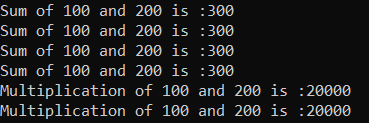##### Understanding Static and Non-Static Constructors in C#:

If we create the constructor explicitly by the static modifier, then we call it a static constructor and the rest of the others are non-static constructors.

The most important point that you need to remember is the static constructor is the first block of code that gets executed under a class. No matter how many numbers of objects you created for the class the static constructor is executed only once. On the other hand, a non-static constructor gets executed only when we created the object of the class and that is too for each and every object of the class.

It is not possible to create a static constructor with parameters. This is because the static constructor is the first block of code that is going to execute under a class. And this static constructor is called implicitly, even if parameterized there is no chance of sending the parameter values.

##### Example to Understand Static and Non-Static Constructors in C#

Let us see an example for a better understanding of the static and non-static Constructors in C#. Please have a look at the below example. In the below example, we have created two constructors. Among the two constructors, one constructor is static and that static constructor is going to be executed first and that constructor is going to be executed only once in its lifetime. Once the static constructor is executed, then the main method starts its execution. Then we created two instances of the Example class and that means the non-static constructor is going to be executed two times. The following example code is self-explained, so please go through the comment lines.

```using System;
namespace StaticNonStaticDemo
{
class Example
{
//Static Constructor
//Executed only once
//First block of code to be executed inside a class
//Before Main Method body start executing, this constructor will execute
static Example()
{
Console.WriteLine("Static Constructor is Called");
}

//Non-Static Constructor
//Executed once per object
//When we create an instance, this constructor will execute
public Example()
{
Console.WriteLine("Non-Static Constructor is Called");
}

//Program Execution will start from the Main method
//But before executing the Main method body, it will
//execute the static constructor
static void Main(string[] args)
{
Console.WriteLine("Main Method Execution Start");
Example obj1 = new Example();
Example obj2 = new Example();
Console.WriteLine("Main Method Execution End");
}
}
}
```
###### Output: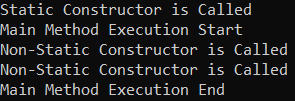##### Static Class in C#:

The class which is created by using the static modifier is called a static class in C#. A static class can contain only static members. It is not possible to create an instance of a static class. This is because it contains only static members. And we know we can access the static members of a class by using the class name.

##### Example to Understand Static Class in C#

Let us see an example for a better understanding of the static class in C#. Please have a look at the below example. As you can see in the below code, we have two classes. The first class TemperatureConverter is a static class and this class contains two static methods. As it is a static class, it can contain only static members. The TestTemperatureConverter is a normal class and from that class, we are calling the static methods by using the static class name.

```namespace StaticNonStaticDemo
{
public static class TemperatureConverter
{
public static double CelsiusToFahrenheit(string temperatureCelsius)
{
// Convert argument to double for calculations.
double celsius = Double.Parse(temperatureCelsius);

// Convert Celsius to Fahrenheit.
double fahrenheit = (celsius * 9 / 5) + 32;

return fahrenheit;
}

public static double FahrenheitToCelsius(string temperatureFahrenheit)
{
// Convert argument to double for calculations.
double fahrenheit = Double.Parse(temperatureFahrenheit);

// Convert Fahrenheit to Celsius.
double celsius = (fahrenheit - 32) * 5 / 9;

return celsius;
}
}
class TestTemperatureConverter
{
static void Main()
{
Console.WriteLine("1. From Celsius to Fahrenheit.");
Console.WriteLine("2. From Fahrenheit to Celsius.");
Console.Write(":");

double F, C = 0;

switch (selection)
{
case "1":
Console.Write("Please enter the Celsius temperature: ");
Console.WriteLine("Temperature in Fahrenheit: {0:F2}", F);
break;

case "2":
Console.Write("Please enter the Fahrenheit temperature: ");
Console.WriteLine("Temperature in Celsius: {0:F2}", C);
break;

default:
break;
}

// Keep the console window open in debug mode.
Console.WriteLine("Press any key to exit.");
}
}
}```
###### Output: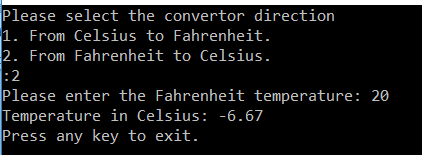In the next article, I am going to discuss Const and Read-Only Variables in C# with Examples. Here, in this article, I try to explain the Static vs Non-Static Members in C# with Examples and I hope you enjoy this Static vs Non-Static Members in C# article. I would like to have your feedback. Please post your feedback, question, or comments about this Static vs Non-Static Member in the C# with Examples article.

### 11 thoughts on “Static vs Non-Static Members in C#”

1.amazing work buddy….

2.Thank you so much. Your explanation is very simple and easy to understand.

3.Simple and clear explanation.

4.useful information and Nice explanation.
Thank you for the article.

5.Sunil Kumar Sharma

Useful information for interview preparation.

6.Superb Article buddy… keep posting like this

7.when to use static constructor in c#? Give more examples

8.Awesome website for learning.. Great work..!!

9.thank you ,your exmplanation simple and clear

10.